Students can download Maths Chapter 4 Geometry Ex 4.1 Questions and Answers, Notes, Samacheer Kalvi 6th Maths Guide Pdf helps you to revise the complete Tamilnadu State Board New Syllabus, helps students complete homework assignments and to score high marks in board exams.

## Tamilnadu Samacheer Kalvi 6th Maths Solutions Term 2 Chapter 4 Geometry Ex 4.1

Question 1.
Fill in the blanks.
(a) Every triangle has at least _____ acute angles.
(b) A triangle in which none of the sides equal is called a _____.
(c) In an isosceles triangle ______ angles are equal.
(d) The sum of three angles of a triangle is ______.
(e) A right-angled triangle with two equal sides is called ______.
Solution:
(a) Two
(b) Scalene Triangle
(c) Two
(d) 180°
(e) Isosceles right-angled triangleQuestion 2.
Match the following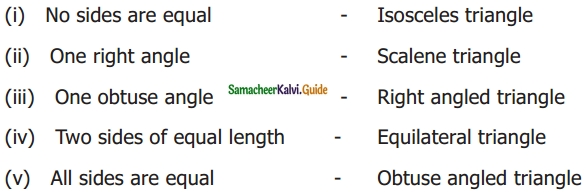Solution:
(i) Scalene triangle
(ii) Right-angled triangle
(iii) Obtuse angled triangle
(iv) Isosceles triangle
(v) Equilateral triangle

Question 3.
In ΔABC, name the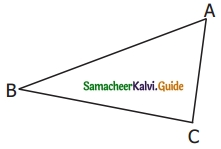a) Three sides: ……….., ………., ……….
b) Three Angles: ………., ………, ……….
c) Three Vertices: ………., ………., ……….
Solution:
(a) $$\overline{\mathrm{AB}}, \overline{\mathrm{BC}}, \overline{\mathrm{CA}}$$
(b) ∠ABC, ∠BCA, ∠CAB or ∠A, ∠B, ∠C
(c) A, B, C

Question 4.
Classify the given triangles based on its sides as scalene, isosceles or equilateral.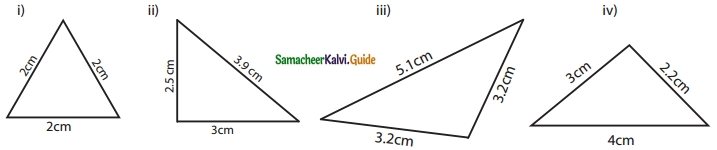Solution:
(i) Equilateral triangle
(ii) Scalene triangle
(iii) Isosceles triangle
(iv) Scalene triangleQuestion 5.
Classify the given triangles based on their angles as acute-angled, right-angled, or obtuse-angled.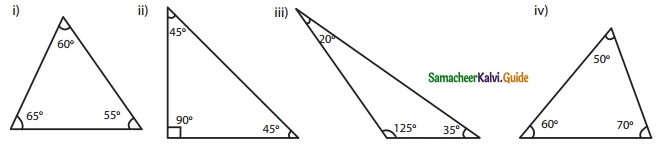Solution:
(i) Acute angled triangle
(ii) Right-angled triangle
(iii) Obtuse angled triangle
(iv) Acute angled triangle

Question 6.
Classify the following triangles based on its sides and angles?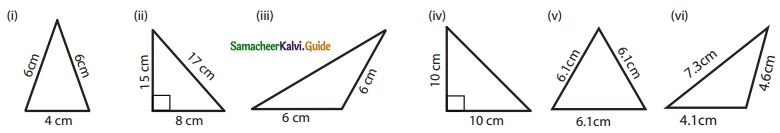Solution:
(i) Isosceles Acute angled triangle
(ii) Scalene Right-angled triangle
(iii) Isosceles Obtuse angled triangle
(iv) Isosceles Right-angled triangle
(v) Equilateral Acute angled triangle
(vi) Scalene Obtuse angled triangleQuestion 7.
Can a triangle be formed with the following sides? If yes, name the type of triangle.
(i) 8 cm, 6 cm, 4 cm
(ii) 10 cm, 8 cm, 5 cm
(iii) 6.2 cm, 1.3 cm, 3.5 cm
(iv) 6 cm, 6 cm, 4 cm
(v) 3.5 cm, 3.5 cm, 3.5 cm
(vi) 9 cm, 4 cm, 5 cm
Solution:
(i) Sum of two smaller sides of the triangle
= 6 + 4 = 10 cm > 8 cm
It is greater than the third side. So, a triangle can be formed a scalene triangle.

(ii) Sum of two smaller sides of the triangle
= 8 + 5 = 13 cm > 10 cm
It is greater than the third side. So, a triangle can be formed a scalene triangle.

(iii) Sum of two smaller sides of the triangle
= 1.3 + 3.5 = 4.8 cm < 6.2 cm
It is not greater than the third side. So, a triangle cannot be formed.

(iv) Two sides are equal.
So, a triangle can be formed. Isosceles triangle.

(v) Three sides are equal.
So, a triangle can be formed equilateral triangle.

(vi) Sum of two smaller sides of the triangle
= 4 + 5 = 9 cm = 9 cm
It is equal to the third side. No, a triangle cannot be formed.Question 8.
Can a triangle be formed with the following angles? If yes, name the type of triangle.
(i) 60°, 60°, 60°
(ii) 60°, 40°, 42°
(iii) 90°, 55°, 35°
(iv) 60°, 90°, 90°
(v) 70°, 60°, 50°
(vi) 100°, 50°, 30°
Solution:
(i) 60°, 60°, 60°
Sum of the angles = 60°+ 60°+ 60° = 180°
A triangle can be formed as an acute-angled triangle.

(ii) 60°, 40°, 42°
Sum of the angles = 60° + 40° + 42° = 142°
A triangle cannot be formed.

(iii) 90°, 50°, 35°
Sum of the angles = 90° + 55° + 35° = 180°
A triangle can be formed Right-angled triangle.

(iv) 60°, 90°, 90°
No, a triangle can not be formed.
A triangle cannot have more than one right angle.

(v) 70°, 60°, 50°
Sum of the angles = 70° + 60° + 50° = 180°
A triangle can be formed as an acute-angled triangle.

(vi) 100°, 50°, 30°
Sum of the angles = 100° + 50° + 30° = 180°
A triangle can be formed as an obtuse-angled triangle.Question 9.
Two angles of the triangles are given. Find the third angle
(i) 80°, 60°
(ii) 75°, 35°
(iii) 52°, 68°
(iv) 50°, 90°
(v) 120°, 30°
(vi) 55°, 85°
Solution:
(i) 80°, 60°
Let the third angle be x.
Sum of the angles = 180°
80° + 60° + x = 180°
140 + x = 180°
x = 180°- 140°
x = 40°
Third angle = 40°

(ii) 52°, 68°
Let the third angle be x.
Sum of the angles = 180°
52° + 68° + x = 180°
120 + x = 180°
180° – 120°
x = 60°
Third angle = 60°

(iii) 75°, 35°
Let the third angle be x.
Sum of the angles 180°
75° + 35° + x = 180°
110 + x = 180°
x = 180° – 110°
x = 70°
Third angle = 70°

(iv) 50°, 90°
Let the third angle be x. Sum of the angles = 180°
50° + 90° + x = 180°
140 + x = 180°
x = 180° – 140°
x = 40°
Third angle = 40°

(v) 120°, 30°
Let the third angle be x.
Sum of the angles = 180°
120° + 30° + x = 180°
150 + x = 180°
x = 180° – 150°
x = 30°
Third angle = 30°

(vi) 55°, 85°
Let the third angle be x.
Sum of the angles = 180°
55° + 85° + x = 180°
140 + x = 180°
x = 180° – 140°
x = 40°
Third angle = 40°Question 10.
I am a closed figure with each of my three angles is 60°. Who am I?
Solution:
Equilateral triangle

Question 11.
Using the given information, write the type of triangle in the table given below.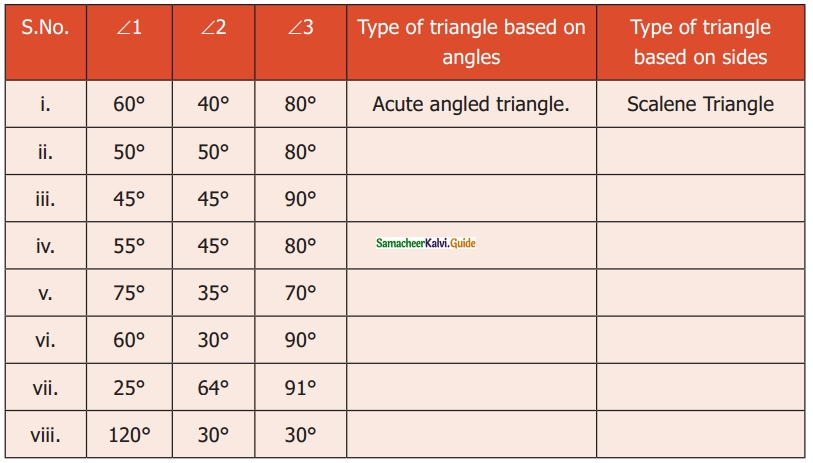Solution:
(ii) Acute angled triangle, Isosceles triangle
(iii) Right-angled triangle, Isosceles triangle
(iv) Acute angled triangle, Scalene triangle
(v) Acute angled triangle, Scalene triangle
(vi) Right-angled triangle. Scalene triangle
(vii) Obtuse angled triangle, Scalene triangle
(viii) Obtuse angled triangle, Isosceles triangleObjective Type Questions

Question 12.
The given triangle is ……….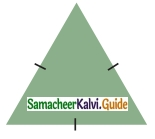(a) a right-angled triangle
b) an equilateral triangle
(c) a scalene triangle
(d) an obtuse-angled triangle
Solution:
(b) an equilateral triangle

Question 13.
If all angles of a triangle are less than a right angle, then it is called _____.
(a) an obtuse-angled triangle
(b) a right-angled triangle
(c) an isosceles right-angled triangle
(d) an acute-angled triangle
Solution:
(d) an acute-angled triangleQuestion 14.
If two sides of a triangle are 5 cm and 9 cm, then the third side is
(a) 5 cm
(b) 3 cm
(c) 4 cm
(d) 14 cm
Solution:
(a) 5 cm

Question 15.
The angles of a right-angled triangle are
(a) acute, acute, obtuse
(c) acute, right, right
(c) right, obtuse, acute
(d) acute, acute, right
Solution:
(d) acute, acute, rightQuestion 16.
An equilateral triangle is
(a) an obtuse-angled triangle
(b) a right-angled triangle
(c) an acute-angled triangle
(d) a scalene triangle
Solution:
(c) an acute-angled triangle